Select PageWe have pretty well established that neither the Hebrew Alphabet, nor the 54-unit shift from it to Phi(ϕ) was arbitrary in the slightest. In order to better understand the nature and relationship of this 54-unit shift, let us visualize these shifting proportions of numbers, not as a line or rectangle but as the circumference and area of a circle.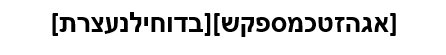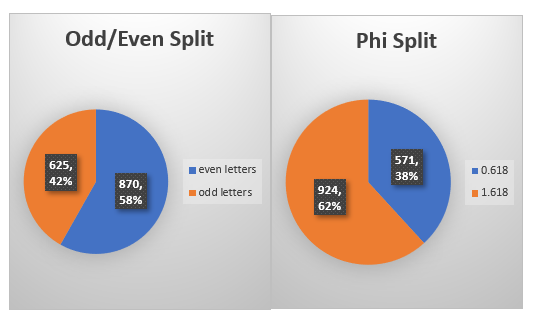We should note that a couple of the amazing properties of Phi(ϕ) are that: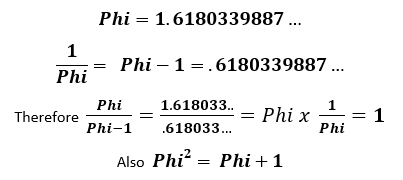By splitting things into the phi proportion, we are splitting it into a proportion of Phi(ϕ) and 1/ Phi(ϕ). One (1) in this case is the whole value of the alphabet, 1495.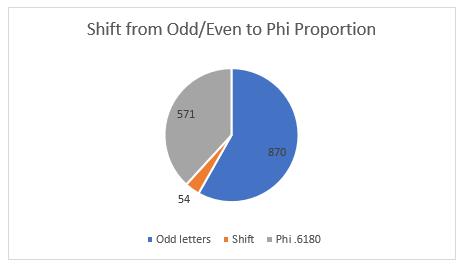Since there are 360֯ in every circle, we can see that:
The Odd Ordinal Letter Proportion represents: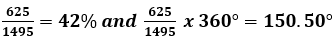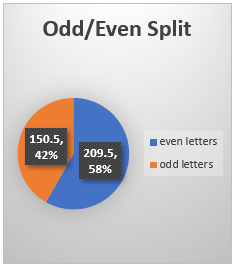The Even Ordinal Letter Proportion represents: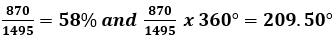The 1/Phi(ϕ) Letter Proportion represents: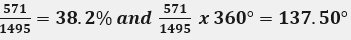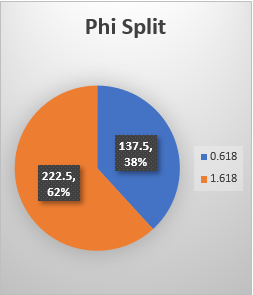The Phi(ϕ) Letter Proportion represents: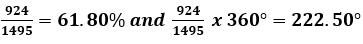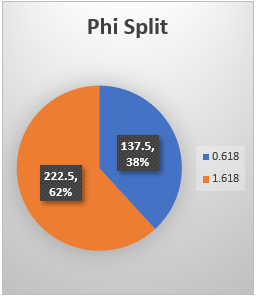This angle 137.50֯ is the Phi(ϕ) angle and is the reason for nature’s efficiency in terms of growth.  If a flower, tree, seashell, galaxy or anything branches outward, one turn of either 137.50֯  or 222.50֯ at a time, as it spirals outward from a central point, it will achieve the most efficient use of time and space possible, thus exerting the least amount of energy necessary for the maximum growth and preservation and propagation of life.Pi is an amazing irrational number of course, but if we were to turn the screws of the spiral according the Pi proportion by using 1/3.14159… instead of 1.618033, as an engine of growth, the result would be 22 spokes of a wheel, analogous to the 22 Letters of the alef-bet, but not the hyper-efficiency as shown in the accompanying picture of the Sunflower.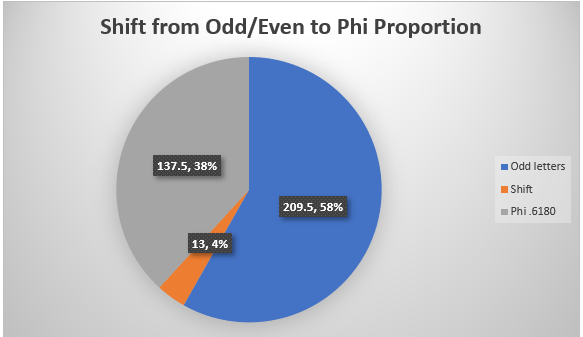The Shift between the Odd/Even Ordinal Letter Proportion and the Phi(ϕ) Proportion represents: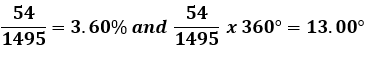Therefore: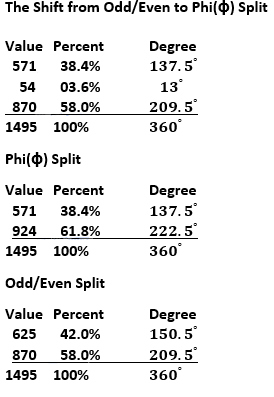All the above charts are simple arithmetic and logical as to why they work out that way. What is not logical is why the greater Phi(ϕ) angle, 222.50֯ less the smaller odd/even angle, 150.50֯, is equal to 72֯ , which was the proportion of the smaller odd/even split to the larger one: (625/870 = 72). There is no mathematical reason that this should happen, especially around such an important number for the Torah.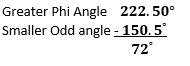The shift of 54 units, or 13֯ caused a 72֯ relationship to appear.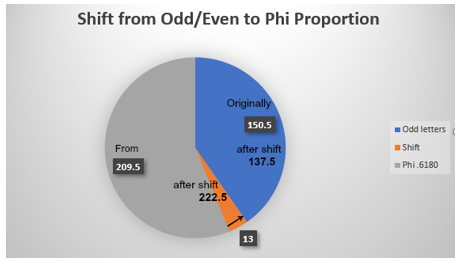Conversely, the lesser Phi(ϕ) angle 137.50֯ plus the odd angle 150.50֯ equals 288֯, which is (360֯ less 72֯).  With the entire Torah based on the number 5, as we have already seen, and with Phi(ϕ) mathematically based on  is it more than curious that 72 is 1/5 of 360, one-fifth of the circle. In Kabbalah 288 is representative of the 288 primordial Holy Sparks that Man was charged with recuperating in order to bring about the final redemption.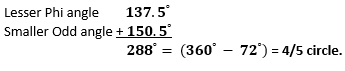Then when we use instead the two larger angles, the larger Even angle and the Greater Phi(ϕ) angle 222.50֯ we get(209.50֯   + 222.50֯ ) = 432֯ = (360֯  + 72֯) and the frequency 432 Hz, derivable from the Torah’s first verse  is a topic for a whole other paper.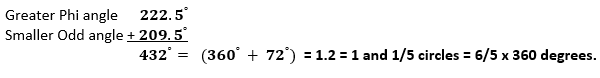This last relationship of 6/5 times 360 degrees is interesting because a circle is dependent on Pi (3.14159…) for its formation utilizing the formula: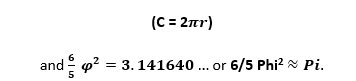This is not causational and may just be coincidental, though it may also be a simple way of showing us that the odd/even ordinal splitting of the alphabet also takes into account the relationship between Phi and Pi.
We only discussed a shift of 54 units, which was equal to a shift of 13 degrees; nevertheless, the sine of an angle of 54֯ is also connected to Phi.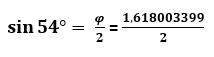What is also not clear at all is why 137.5/924 = 13%. Why does the resultant angle  divided by the value of the larger section (924) also equal 13? If you are wondering what the relationship is between 137.50֯ and 924 units, so are we because one does not exist.
As for the separate relationship between 137.5 and 5 cubits, we will get into that in a later section.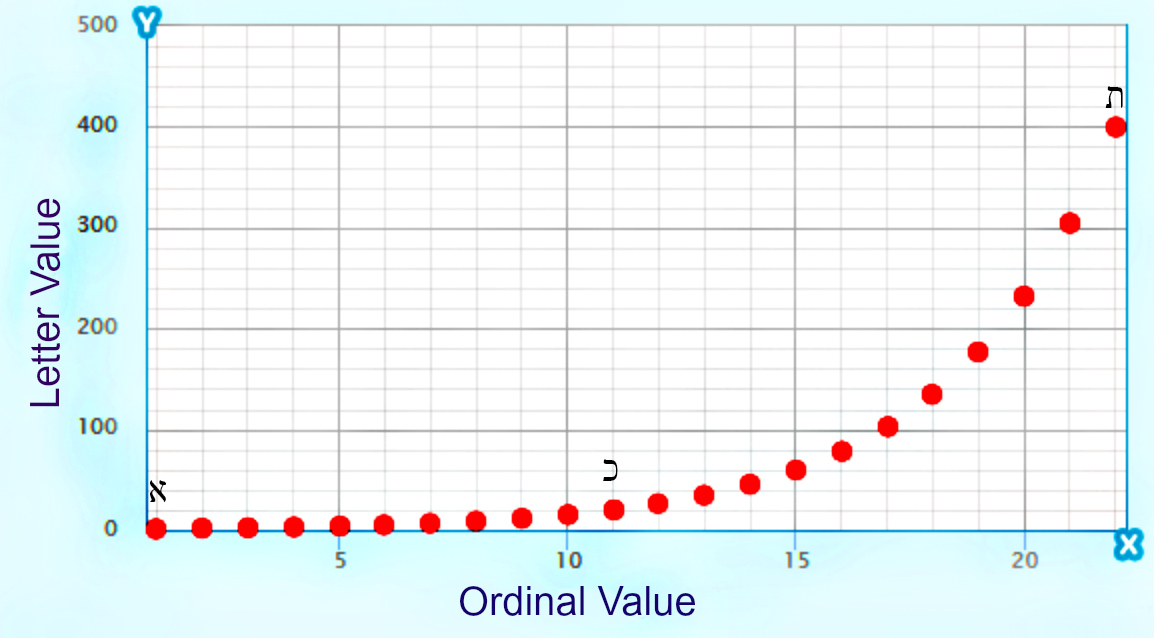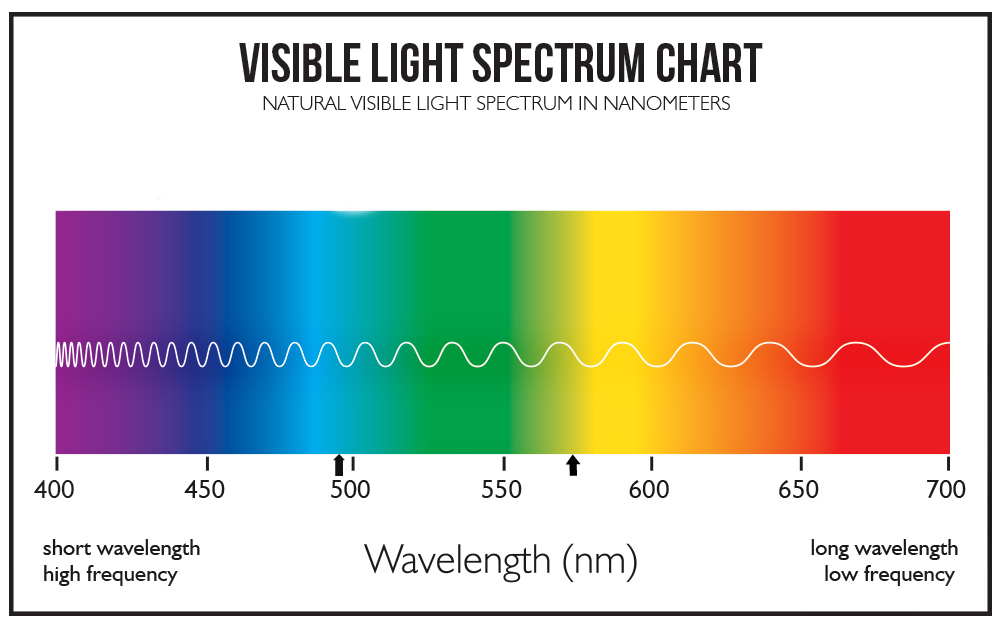This may be a good point to remind ourselves that the numbers associated with the alef-bet and carved in stone for millennia are more complicated than that. Like the location of the electrons encircling the nuclei of the atom, they are the probabilities within the energetic bandwidth along the exponential curve given by 1.313, and like the 4th word of the Torah, they are anchored by 1 and 400.
There is no reason other than divine intent. What all these anglerelationships are telling us is that placing the odd/even ordinal split into a circular form and comparing it with Phi(ϕ) is not only valid, but was part of the initial intent.  It is also telling us that all along we have been lead to the number 13.
Do you believe in coincidences? That 1st chapter in Bamidbar that split the Torah into the Phi proportion in terms of each of its main elements (words, letters and verses), the one with 54 verses, delineated 13 Tribes.

## ONE

So why is the 54-shift being equivalent to 13֯ significant? Because the number 13, as a gematria value has always been universally recognized as the numerical value of Ahava (אהבה), meaning love and Echad (אחד), meaning One.
In Zechariah 14:7 we learn that there will come a day when the darkness will be no more, the night and evening will be no more and there will only be light; this is called “One” day (יוֹם-אֶחָד) , in which all will be restored as before anything was split, before the light was split. It is a reset and a redo because the last time around at the end of Day one (יוֹם-אֶחָד) there was already division of Light and darkness (Bereshit 1:4-5).  This One day, or day of perpetual unity, shall be known as the Lord’s Day. Hashem’s Day.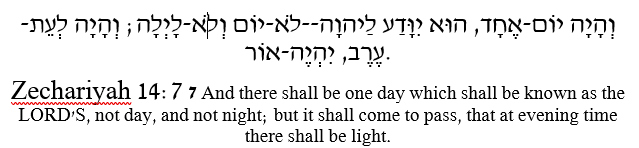In Zechariah 14:9 we have one of the more famous refrains for the Kabbalists, one weaved into our prayer books. “On that day shall He and His Name be One/He and His Name are One.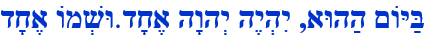We posit that this is the “One” that the 13֯ shift is hinting at.  This profound 7-word 26-letter verse that connects to the same pure Oneness concept of the 6-word first verse of the Shema and the Torah’s 7-word first verse, has very interesting properties. First of all, the 7 final letters add up to 625, or the square root of the Torah’s main elements, and the final letters of the last 6 words sum to 25, evocative of the Odd Ordinal Number splitting of the alef-bet.
The rest of the letters in the verse equal 444, the gematria of the important Torah phrase “from generation to generation (לדר־ודר).” The number 444 is also approximately equal to One(1) continually divided by the Bell Prime Indices.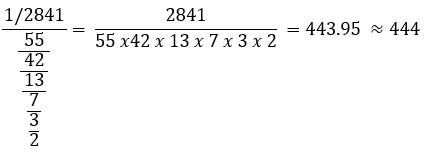Next, the 3 central words (הַהוּא, יִהְיֶה יְהוָה) add up to 73, as in the Torah’s first verse.  Also, at 618, the value of the verse’s first word is evocative of phi (.6180). Moreover, there are 26 letters in the verse, reflecting the Tetragrammaton (יהוה), the central word in the verse. Meanwhile, the 10 letters in Echad Ushmo Echad (אחד־ושמו־אחד) add up to 378, the sum of the numbers from 127 as in 33 and the 27 Letters of the complete Hebrew Alef-bet, whose total gematria is 4995 or (5000 -5) or 5(1000-1). This is evocative of the 1000 unit concept of the 72 Triplets, the 1000 letters of the Shema, and the 1000-unit different between the first verse and the 42-Letter Name, coupled with the concept of One(1), and the Unit of 5 at the core of Phi and the Torah. And that is just the beginning.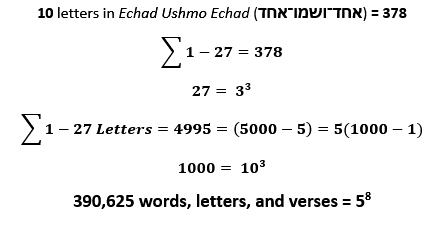Speaking of Shema prayer, this “One” is the same “One” in its first verse.   Devarim 6:4 “Hear, O Israel, the Lord our G-d the Lord is One.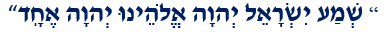The Lord is One is a huge concept that includes a wholeness before anything was split, any division. You can just look around us today and see the anger, hatred, havoc and strife that division sews.  All the technology embedded in the Torah is designed to focus us on reuniting, on unity with the Creation and the Creator, with the Light before the darkness. Oneness. If you are not a conscious part of the matrix (virtual or real, simulation or physical) you just a prisoner of it. In order to get there, to the state of Oneness, there is a shift we must make.  First, we must awaken.  The Torah is providing us with all the clues how to make that shift, how to dial into that frequency of Oneness, how to awaken.
Oneness is One with the Creator or Creative forces of the greater universe. It is not singing kumbaya because you received a mass twitter feed or shouting “we are one” at a rally or staged event. That is manipulation. Oneness awakens within. This is why there was 3 days of fasting before the unity of the Jews in the Megillat Ester. They needed to separate from physicality. It is the same principle on the Day of atONEment.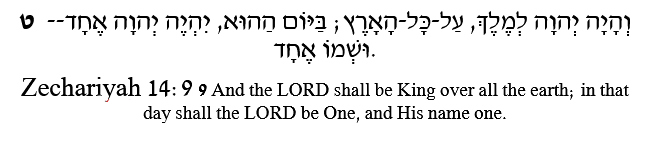If you recall, we stated early on, before we split the alef-bet in two, that the Hebrew alphabet was designed according to an exponential scale based on a factor of 1.313, which is the inverse of the logarithmic scale, so how apropos is it that the logarithm of 13 is 1.11, mimicking the numerical value (111) of the first letters of the alphabet Alef(אלף).Phi(ϕ) is a very special mathematical constant and like Pi, if it did not exist, neither would we. Our physicality could not exist without the scaffolding that maintains it anymore than a building can exist without the metal or wood framing that gives it its basic shape. Before we reveal Phi’s absolute relationship with the number 1, we want to remind you about the alphabet and Phi(ϕ) splits with regards to 1.
Adding the digits in the odd/even ordinal split:

6 + 2 + 5 + 8 + 7 + 0 = 28  2 + 8 = 1 (One)

Adding the digits in the Phi(ϕ) split:

5 + 7 + 1 + 9 + 2 + 4 = 28  2 + 8 = 1 (One)

Adding the digits of the total value of the alef-bet plus the shift of 54: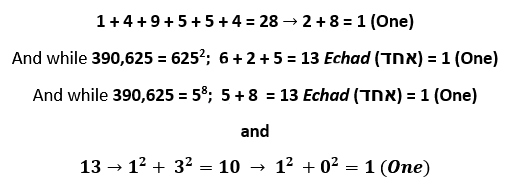The number 13 reduces to 1 (One) in yet another way. It is part of the set of “happy” numbers, whose digits when squared then added and the process continually repeated, eventual reduce to 1. Other happy numbers are 7, 10, 28, 70
Part of the beauty and efficiency of Phi (ϕ) is its source: 1 (One). Phi (ϕ) can be generated by utilizing 1 single number, the number 1 (One). It is true that any number can be generated by adding 1, for example 5 is (1 + 1 + 1 + 1 +1) but that is cheating a bit and Phi (ϕ) is an irrational number (1.618033887…) so adding 1+1 does not work; it only works with whole numbers. That said, the continual fraction expansion of Phi (ϕ), in other words, Phi (ϕ) as an infinite fraction is: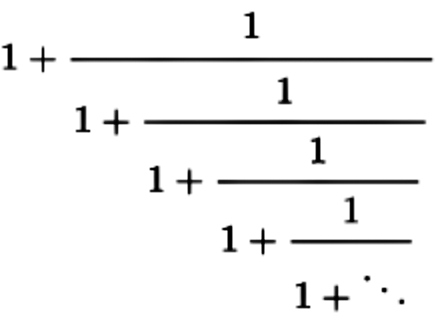Also: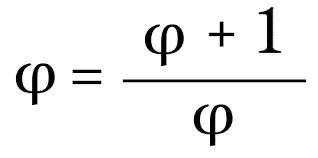Also: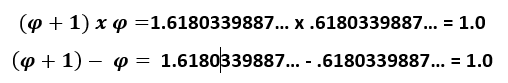We can appropriately regard Phi (ϕ) as derivative of 1 (One).  And the 13-degree shift in the odd/even ordinal alef-bet split toward the Phi () split as a shift towards 1 (One).

### The Shift is a Shift Towards One

Every irrational number can easily be written as an infinite fraction. Of them all, Phi (ϕ) is the most difficult and is unique in that it has exactly 1 numeral. Every irrational number serves a specific purpose in the universe. Every number serves a purpose in the Torah; every number can be a heavenly portal if approached from the right paths. It appears as if Phi ) has a direct route.
As strange as it is, the Phi (ϕ) split number 924 is found at the center of the 13th line of Pascal’s triangle.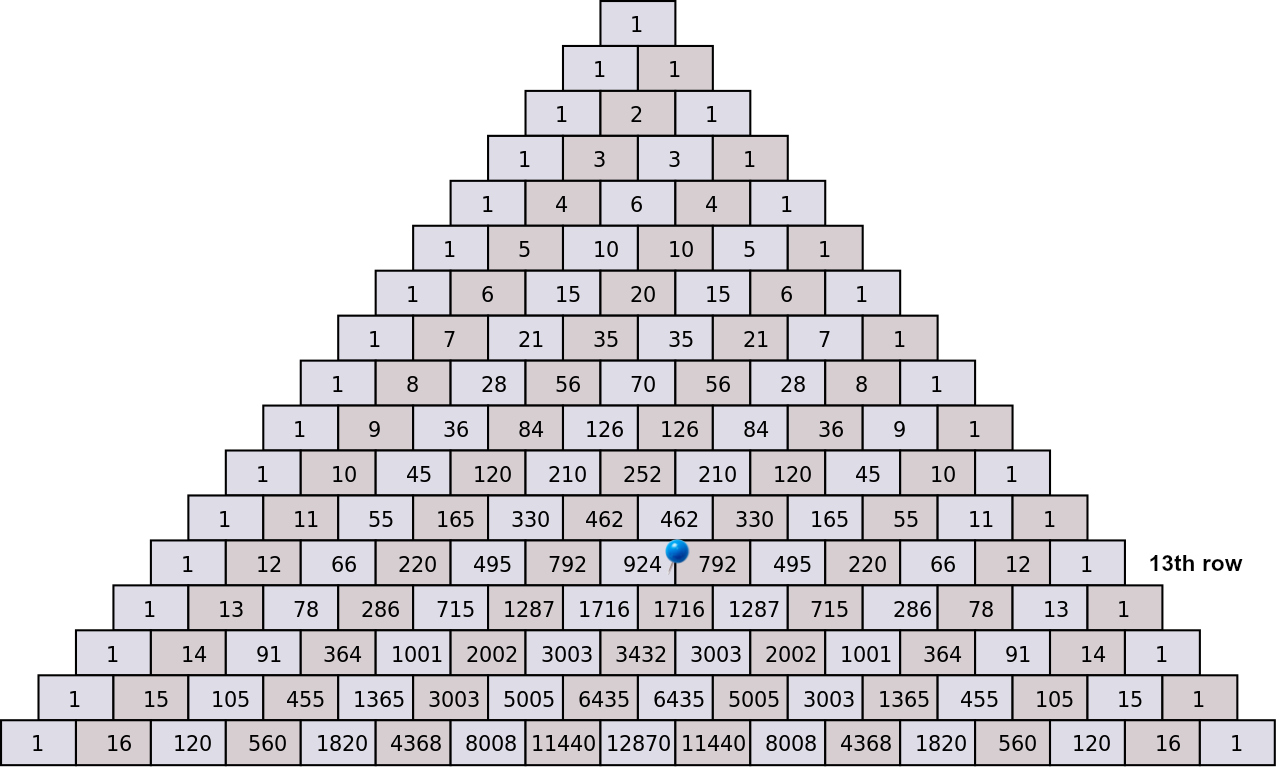There are numerous Torah numbers embedded in the palindromic Pascal’s pyramid, but the beauty in it lies in its simplicity, and that so many mathematical series and functions can be generated from it so easily: the Fibonacci number series that is directly proportionate to Phi (ϕ); the sequence of 2n by adding up the numbers in the rows; the sequence of 11n by using the small gematria of each row’s number; and so many other sequences.

## The Pilots

The Name Moshe (משה) breaks down in terms of its ordinal value into Mem(מ), 13, and Shin-Hei(שה), 26, or Adonai Echad (אחד  יהוה). The assignation, Moshiach (משיח) breaks down in terms of its ordinal value into Mem(מ), 13, and Shin-Yud-Chet(שיח), 39, or Echad Adonai Echad  (אחד  יהוה  אחד).
Is there any doubt behind the guiding principles of the launch codes?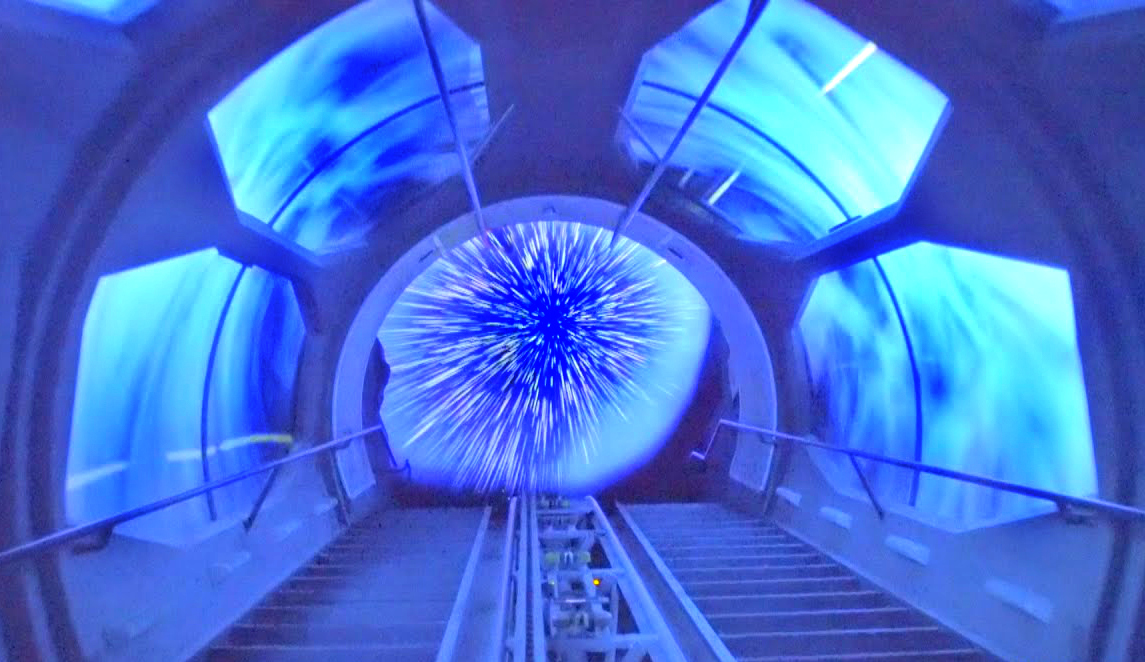So how do we get a ticket to this hyperspace freedom adventure ride? The cost of the ticket is different for all of us and is wholly dependent on our individual attachment to physicality. The price of the ticket is free, but the height bar is set at “Awoken.” That’s why we refer to the tzaddikim as giants. If you cannot let go, you cannot board—it is as simple as that.
And in case we think that the shift will be made for us from above rather than of our own volition and as a result of our own actions, we need only look to the hint provided in the Torah’s first verse once again. The ordinal value of the final word, “the earth (הארצ)” is 26 + 18 = 44. The good news is the that the message also reads “G-d Lives (יח יהוה)” and Phi (ϕ)2 as 2.618. Which leads us to our next section where we examine possible launch codes and sequences, metaphorically and metaphysically, and explore one of the tools given to us to foster that awakening.